Скачать презентацию Measurement Perimeter Area Applications Objectives By April Rodriguez

4f93582a84e584f64fc9576af378e58c.ppt

• Количество слайдов: 32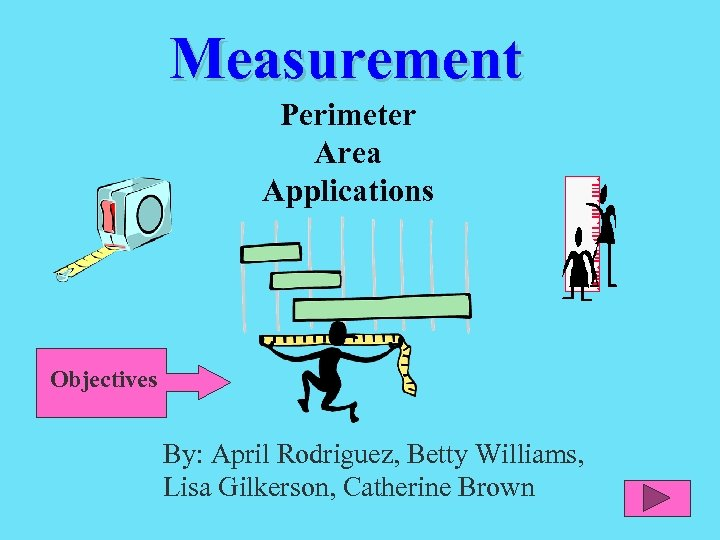Measurement Perimeter Area Applications Objectives By: April Rodriguez, Betty Williams, Lisa Gilkerson, Catherine Brown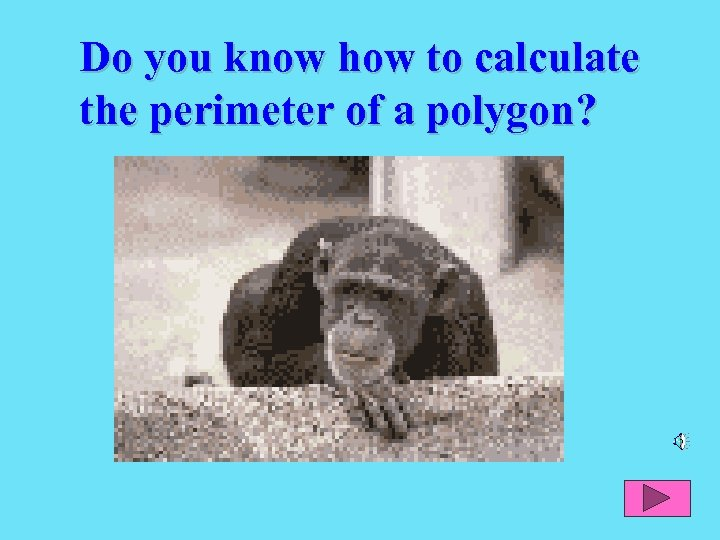Do you know how to calculate the perimeter of a polygon?Polygons come in all shapes and sizes.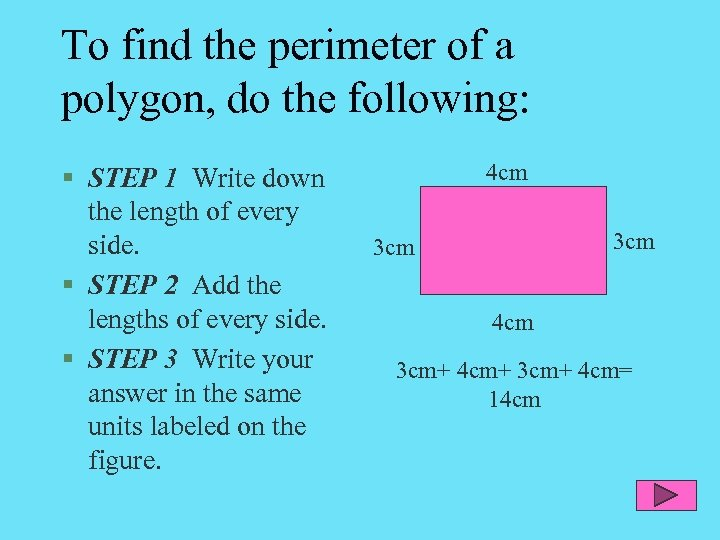To find the perimeter of a polygon, do the following: § STEP 1 Write down the length of every side. § STEP 2 Add the lengths of every side. § STEP 3 Write your answer in the same units labeled on the figure. 4 cm 3 cm+ 4 cm+ 3 cm+ 4 cm= 14 cm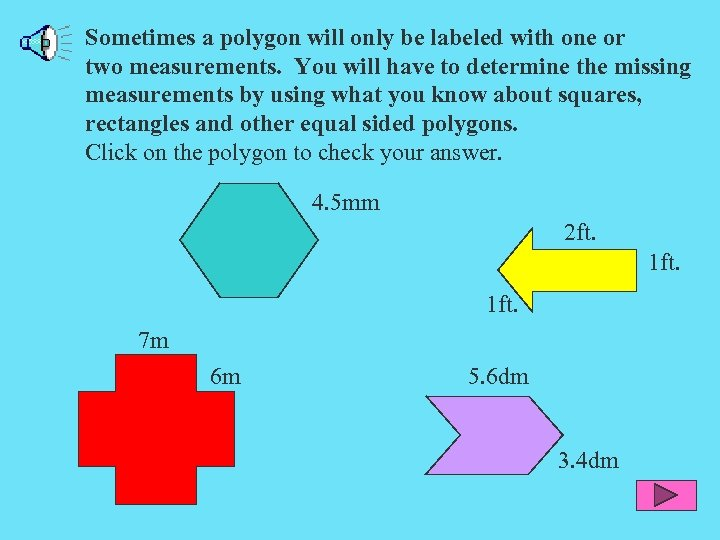Sometimes a polygon will only be labeled with one or two measurements. You will have to determine the missing measurements by using what you know about squares, rectangles and other equal sided polygons. Click on the polygon to check your answer. 4. 5 mm 2 ft. 1 ft. 7 m 6 m 5. 6 dm 3. 4 dm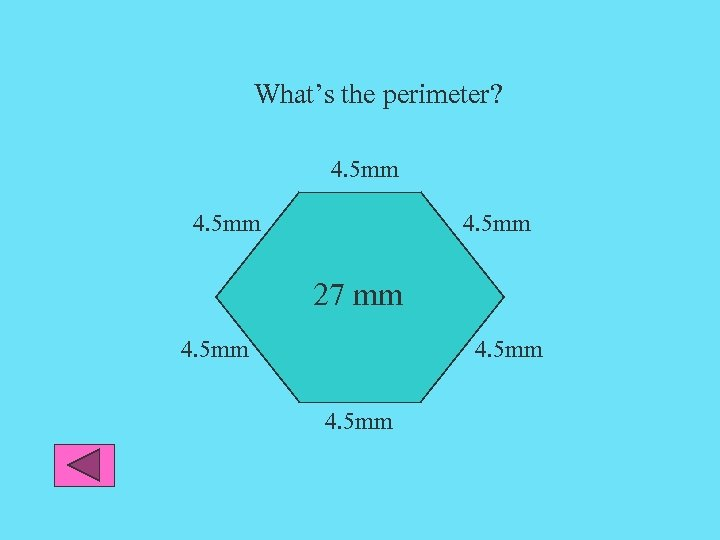What’s the perimeter? 4. 5 mm 27 mm 4. 5 mm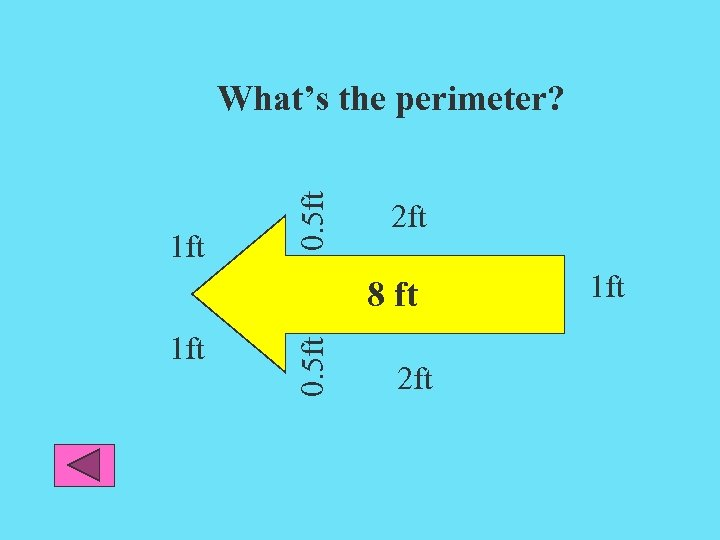1 ft 0. 5 ft What’s the perimeter? 2 ft 1 ft 0. 5 ft 8 ft 2 ft 1 ft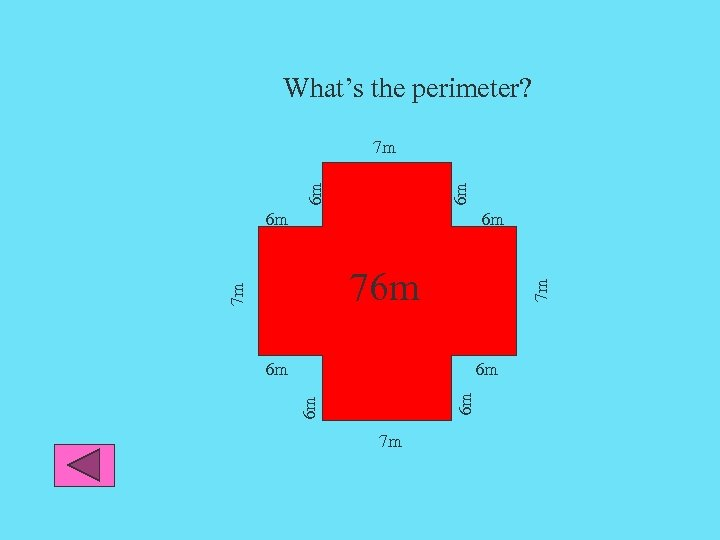What’s the perimeter? 6 m 6 m 7 m 7 m 76 m 6 m 6 m 7 m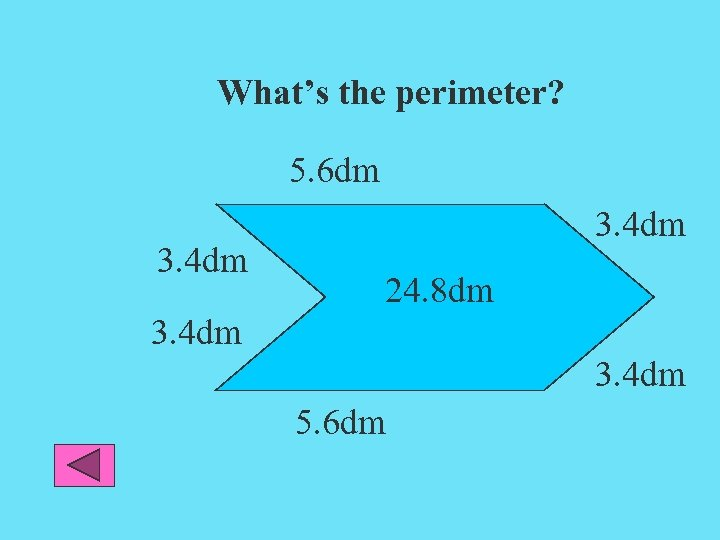What’s the perimeter? 5. 6 dm 3. 4 dm 24. 8 dm 3. 4 dm 5. 6 dm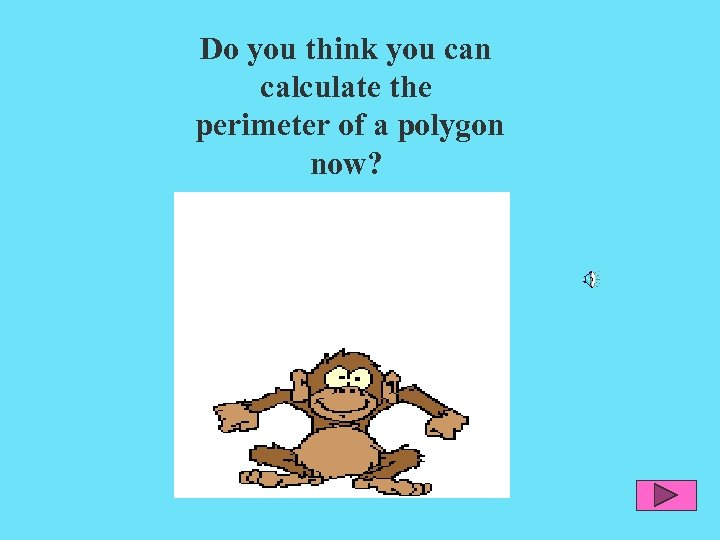Do you think you can calculate the perimeter of a polygon now?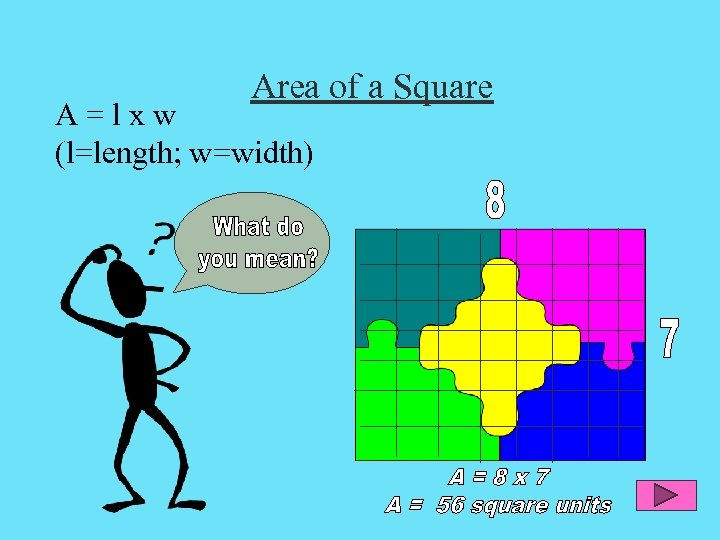Area of a Square A=lxw (l=length; w=width)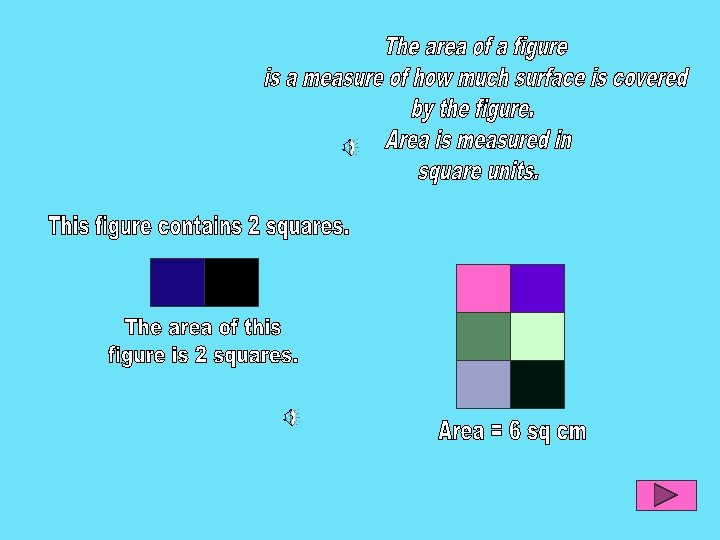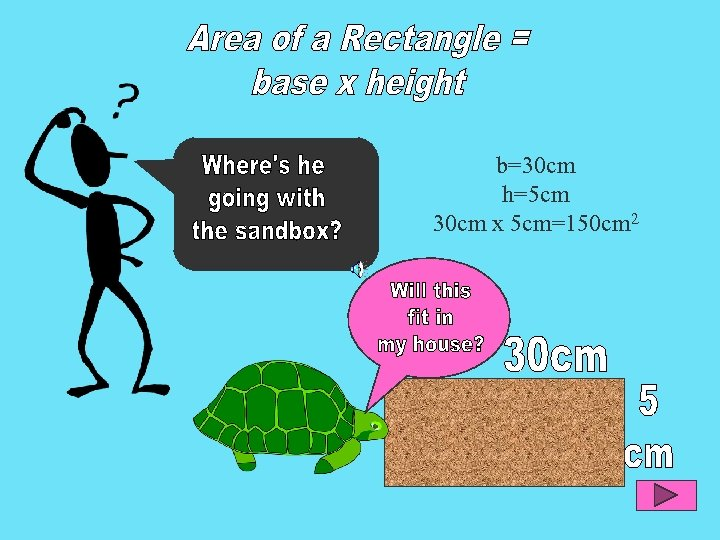b=30 cm h=5 cm 30 cm x 5 cm=150 cm 2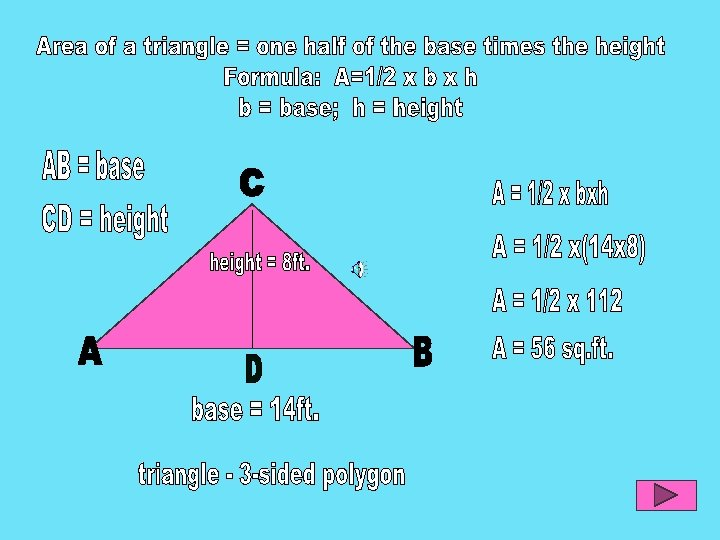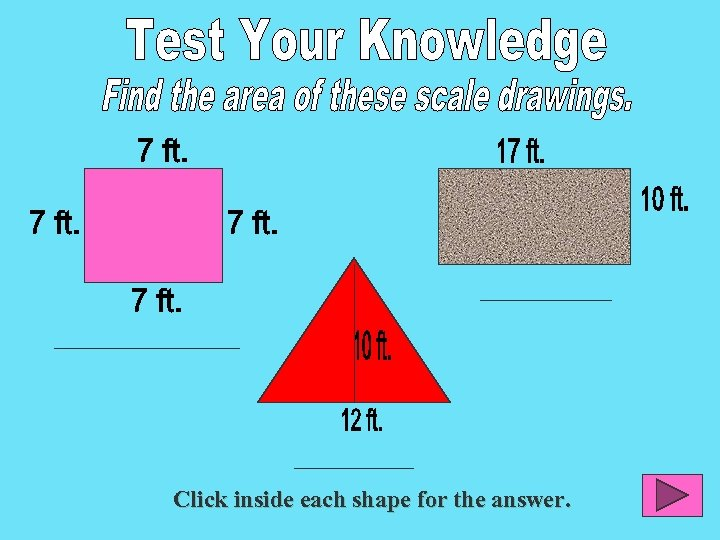Click inside each shape for the answer.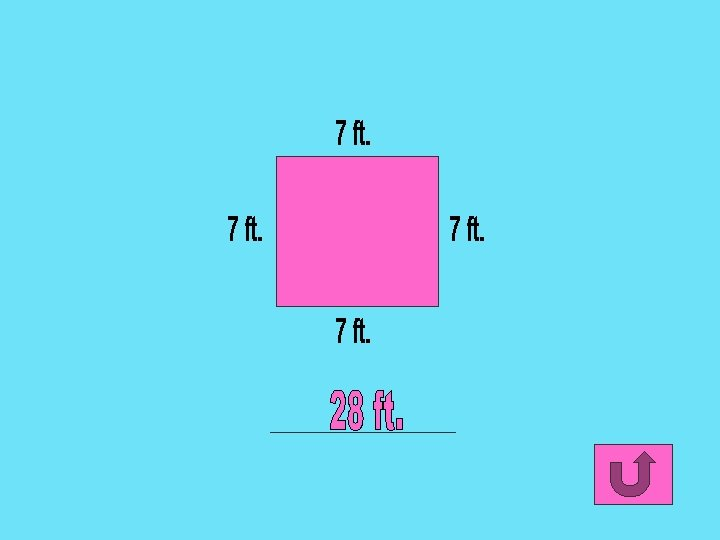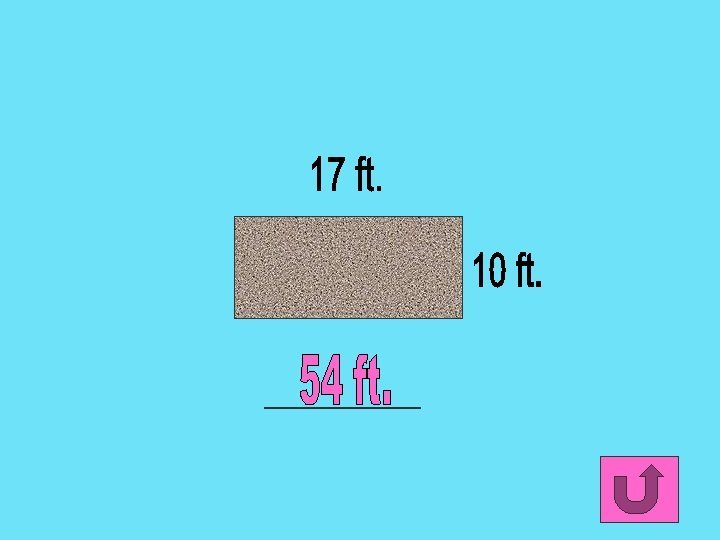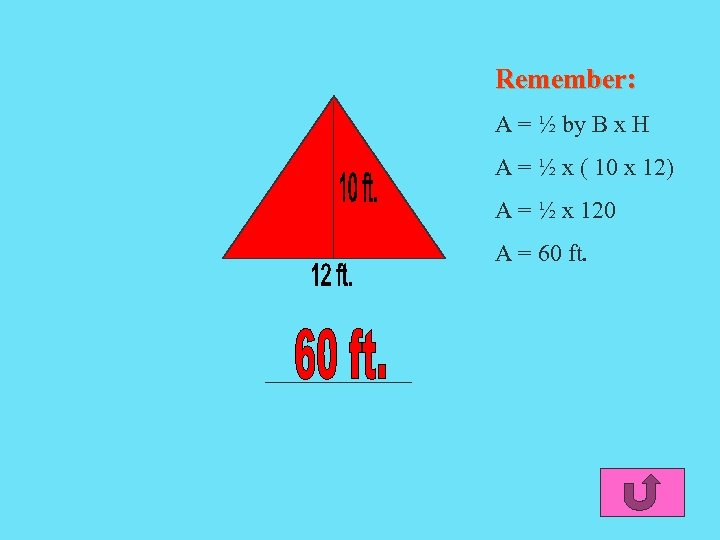Remember: A = ½ by B x H A = ½ x ( 10 x 12) A = ½ x 120 A = 60 ft.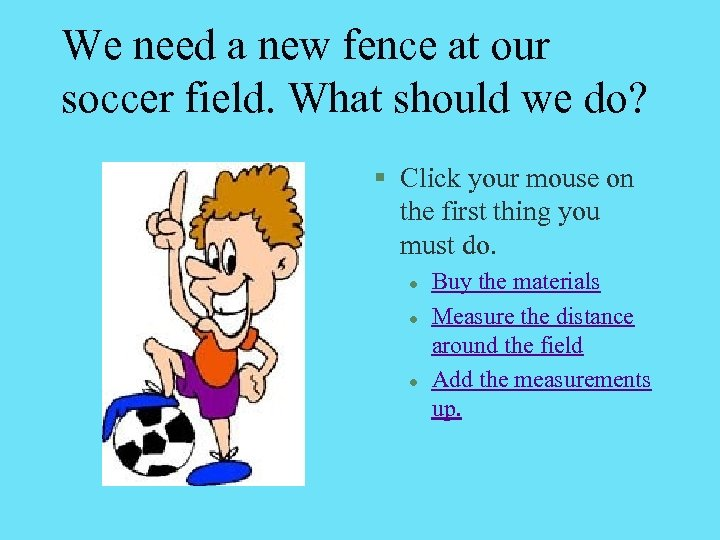We need a new fence at our soccer field. What should we do? § Click your mouse on the first thing you must do. l l l Buy the materials Measure the distance around the field Add the measurements up.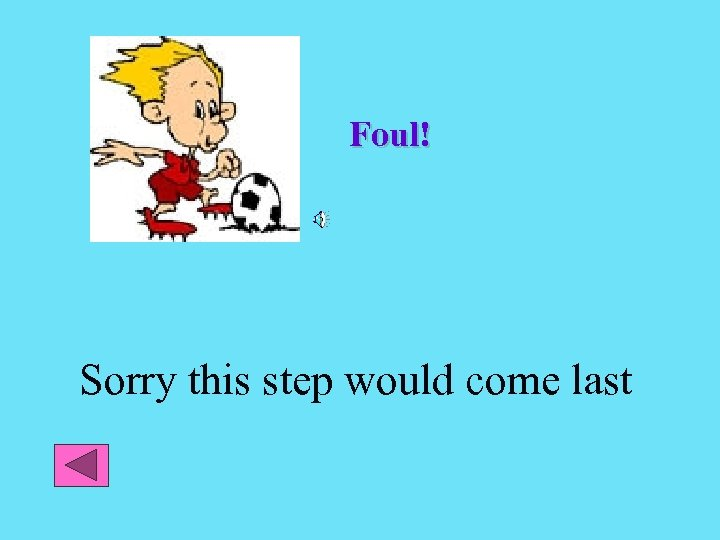Foul! Sorry this step would come last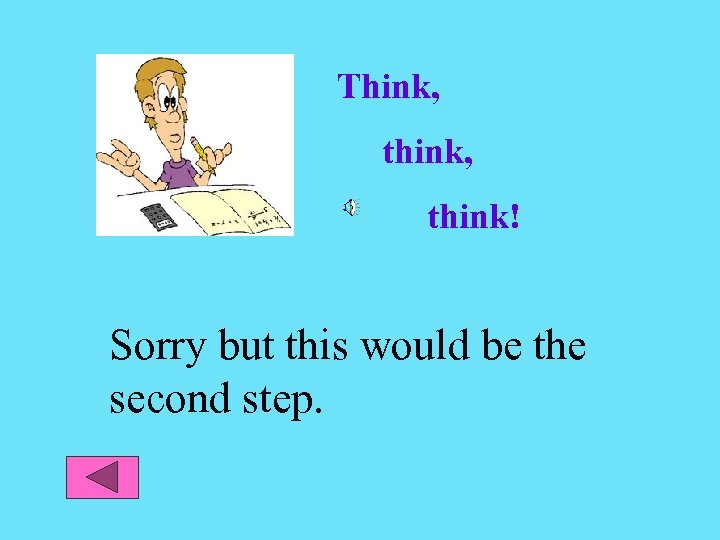Think, think! Sorry but this would be the second step.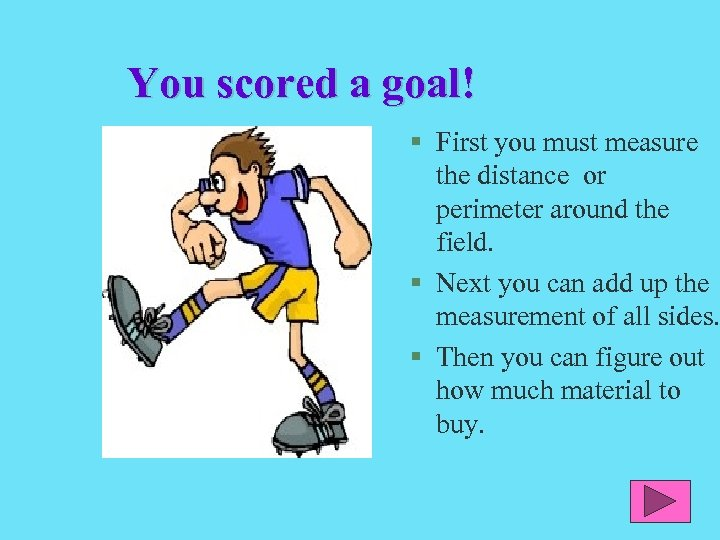You scored a goal! § First you must measure the distance or perimeter around the field. § Next you can add up the measurement of all sides. § Then you can figure out how much material to buy.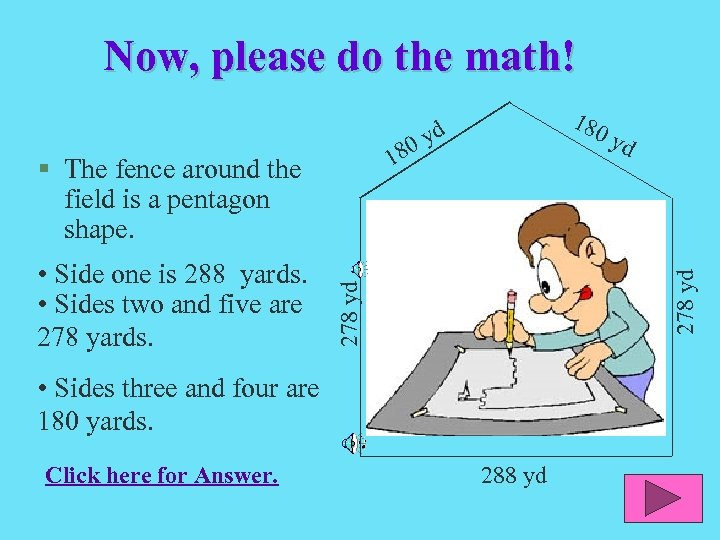Now, please do the math! 1 • Side one is 288 yards. • Sides two and five are 278 yards. 278 yd § The fence around the field is a pentagon shape. • Sides three and four are 180 yards. Click here for Answer. yd 278 yd 80 180 yd 288 yd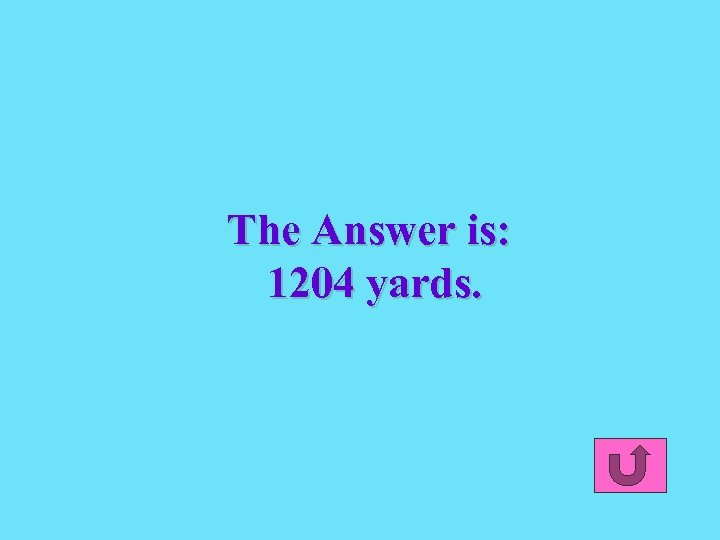The Answer is: 1204 yards.Although I love soccer, my summer job is working at RFK, the old Washington Redskins Stadium. We have to plant new grass. Whew, we have a lot of yardage to cover! Boy, knowing about perimeter and area would really help me right now!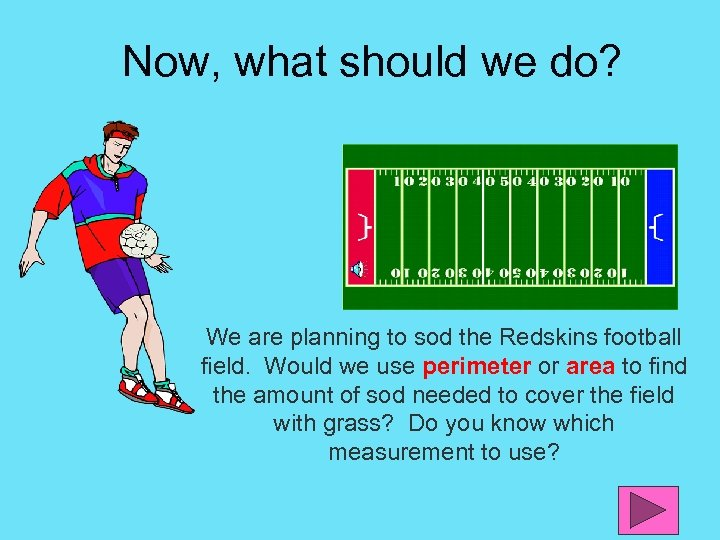Now, what should we do? We are planning to sod the Redskins football field. Would we use perimeter or area to find the amount of sod needed to cover the field with grass? Do you know which measurement to use?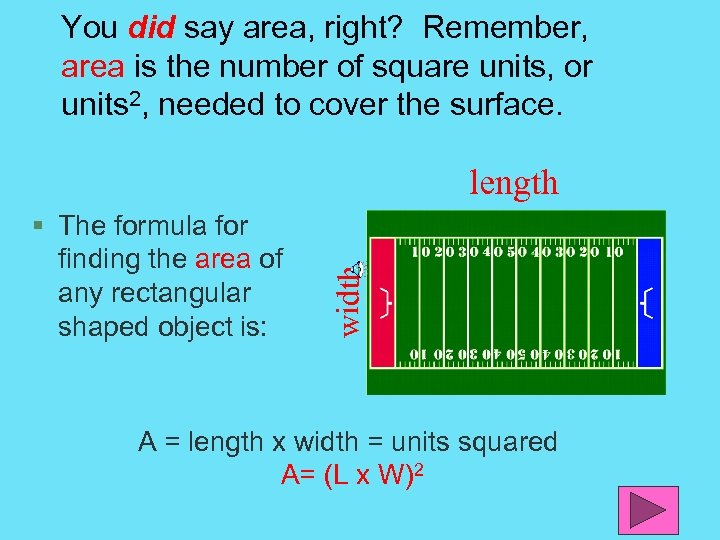You did say area, right? Remember, area is the number of square units, or units 2, needed to cover the surface. § The formula for finding the area of any rectangular shaped object is: width length A = length x width = units squared A= (L x W)2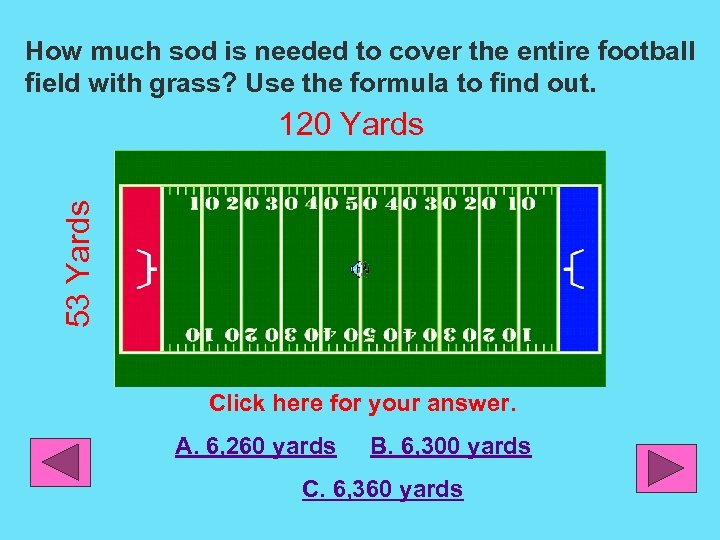How much sod is needed to cover the entire football field with grass? Use the formula to find out. 53 Yards 120 Yards Click here for your answer. A. 6, 260 yards B. 6, 300 yards C. 6, 360 yards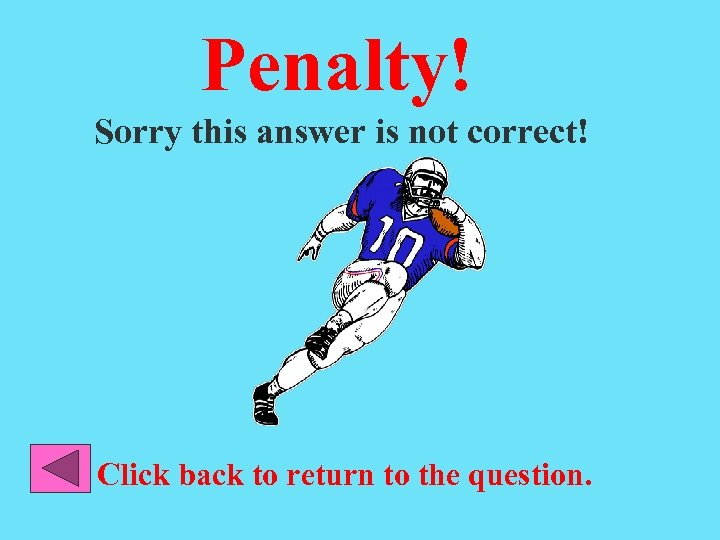Penalty! Sorry this answer is not correct! Click back to return to the question.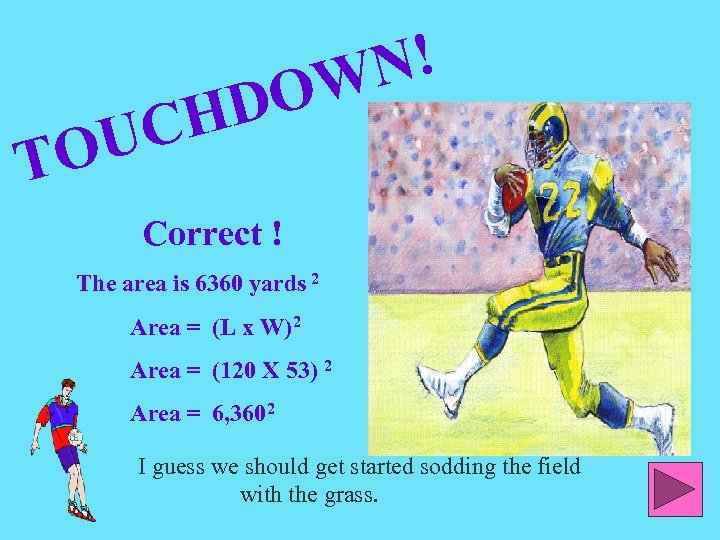TO HD UC N! OW Correct ! The area is 6360 yards 2 Area = (L x W)2 Area = (120 X 53) 2 Area = 6, 3602 I guess we should get started sodding the field with the grass.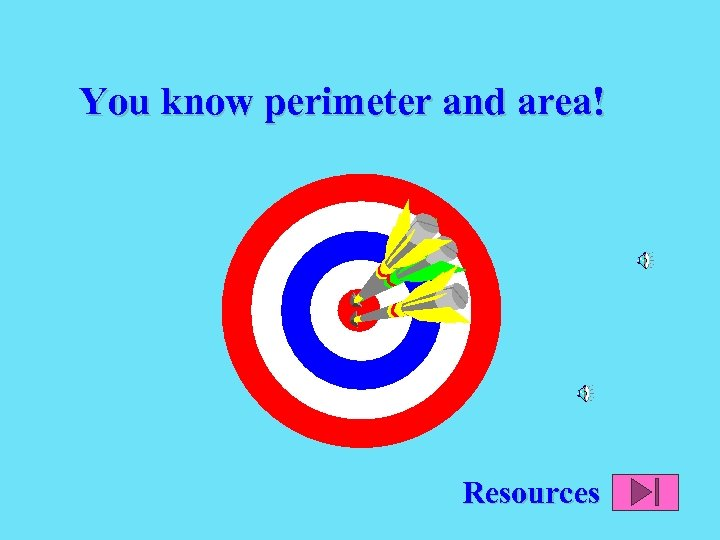You know perimeter and area! ResourcesPlease use the following resources to make sure you can find the perimeter and area of polygons http: //www. funbrain. com/cgi-bin/poly. cgi? A 1=s&A 2=1&A 15=1&INSTRUCTS=1 http: //www. mathgoodies. com/lessons/toc_vol 1. shtm http: //www. indiana. edu/~atmat/units/area_perimeter/area_intro. htm